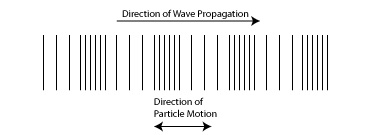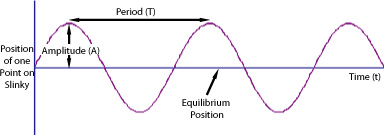Mechanics

# Waves and Wave Motion: Describing Waves

by Nathaniel Page Stites, M.A./M.S.

On July 17, 1998, three huge waves – "tsunamis" – up to 15 meters high struck the north coast of Papua New Guinea, killing at least 2,200 people. A major earthquake, itself consisting of waves traveling through the Earth, triggered an underwater landslide that created the tsunamis. Radio stations reported the disaster by transmitting electromagnetic radio waves to listeners around the world. Listeners were able to hear the news transported by sound waves created by their radios.

Waves of one form or another can be found in an amazingly diverse range of physical applications, from the oceans to the science of sound. Put simply, a wave is a traveling disturbance. Ocean waves travel for thousands of kilometers through the water. Earthquake waves travel through the Earth, sometimes bouncing off the core of the Earth and making it all the way back to the surface. Sound waves travel through the air to our ears, where we process the disturbances and interpret them.

## Ancient wave theories

Much of our current understanding of wave motion has come from the study of acoustics. Ancient Greek philosophers, many of whom were interested in music, hypothesized that there was a connection between waves and sound, and that vibrations, or disturbances, must be responsible for sounds. Pythagoras observed in 550 BCE that vibrating strings produced sound, and worked to determine the mathematical relationships between the lengths of strings that made harmonious tones.

Scientific theories of wave propagation became more prominent in the 17th Century CE, when Galileo Galilei (1564-1642) published a clear statement of the connection between vibrating bodies and the sounds they produce. Robert Boyle, in a classic experiment from 1660, proved that sound cannot travel through a vacuum. Isaac Newton published a mathematical description of how sound travels in his work Principia (1686). In the 18th Century, French mathematician and scientist Jean Le Rond d'Alembert derived the wave equation, a thorough and general mathematical description of waves, which laid the foundation for generations of scientists to study and describe wave phenomena.

Comprehension Checkpoint

Our understanding of wave motion began with the study of

## Wave basics

Waves can take many forms, but there are two fundamental types of waves: "longitudinal" and "transverse" (see Figures 1 and 2). Both of these wave types are traveling disturbances, but they are different because of the way that they travel. As a wave travels through a medium, the particles that make up the medium are disturbed from their resting, or "equilibrium" positions. In a longitudinal wave, the particles are disturbed in a direction parallel to the direction that the wave propagates. A longitudinal wave consists of "compressions" and "rarefactions" where particles are bunched together and spread out, respectively (see Figure 1). For another view of this type of wave, take a look at the longitudinal wave video clip below. In a transverse wave, the particles are disturbed in a direction perpendicular to the direction that the wave propagates. The transverse wave video clip below provides a dynamic visualization of this type of wave. After either type of wave passes through a medium, the particles return to their equilibrium positions. Thus, waves travel through a medium with no net displacement of the particles in the medium.Figure 1: A longitudinal wave, made up of compressions – areas where particles are close together – and rarefactions – areas where particles are spread out. The particles move in a direction that is parallel to the direction of wave propagation.Figure 2: A transverse wave. The particles move in a direction that is perpendicular to the direction of wave propagation.

Sound waves are examples of longitudinal waves: the individual particles (air molecules) vibrate back and forth in the direction that the sound is traveling. An example of a transverse wave is the classic sports arena phenomenon known as "The Wave." As the wave travels around the stadium, each spectator stands up and sits down. Thus, the displacement of the "particles" is perpendicular to the direction the wave travels. Many other waves, such as ocean waves or Rayleigh Surface Waves are combinations of longitudinal and transverse wave motion.

Comprehension Checkpoint

Particles bunch together and spread out in _____ waves.

## Describing waves

The waves we described above are all examples of 'periodic waves,' in that they involve a cyclical pattern of motion. Waves travel through space and time, and can be described in terms of their characteristics in both of these dimensions. Imagine a Slinky®, a toy that consists solely of a long, loosely coiled piece of metal or plastic. By shaking one end of the Slinky® up and down in a periodic fashion, it is possible to produce a transverse wave, as shown in the figures below.

### Amplitude and wavelength

Figure 3 represents a snapshot of a Slinky®, such as the one in the transverse wave video clip, as it is vibrating. The vertical axis represents the vertical position of the Slinky®, and the horizontal axis represents its horizontal position. As indicated in the figure, the amplitude (A) of the wave is the maximum displacement of a particle from its equilibrium position – or the height of the wave. The length of the wave is the wavelength (λ), and is simply the length of one cycle of the wave. In the figure, the wavelength is shown as the distance between two successive wave crests. The wavelength can also be measured between successive troughs, or between any two equivalent points on the wave. Both the amplitude and the wavelength of a wave are commonly measured in meters.Figure 3: A view of a Slinky® at a particular moment in time.

Comprehension Checkpoint

The height of a wave is know as its

### Wave period

Figure 4 is a graph of the displacement of one point on the Slinky® as a function of time. The amplitude of the wave is still the same measurement as before – the maximum displacement of the point from its equilibrium position. The wave period (T) is the time (measured in seconds) required for the point to complete one full cycle of its motion, from its highest point to its lowest and back again.Figure 4: The motion of one point on a Slinky® as it travels through time.

### Wave frequency

The frequency of a wave (f) (not indicated in the figure) is a measure of how frequently the point completes one cycle of its motion. In other words, the frequency is the number of wave cycles completed by one point along the wave in a given time period. The frequency of a wave is related to the period of a wave by the following equation:

$$f = 1 T$$

Where f is the frequency and T is the wave period. The frequency is measured in cycles per second, or hertz (Hz). If the wave period is 10 seconds (that is, it takes 10 seconds for the wave to complete one cycle), then the frequency is 0.1 Hz. In other words, the wave completes 0.1 cycles every second.

Comprehension Checkpoint

A frequency of 0.1 Hz means that a wave completes _____ cycles every second.

### Wave speed

Remember that a wave is a traveling disturbance. Wave speed is a description of how fast a wave travels. The speed of a wave (v) is related to the frequency, wave period, and wavelength by the following simple equations:

$$v = λ T$$

$$v = λ f$$

where v is the wave speed, λ is the wavelength, T is the wave period, and f is the frequency. Wave speed is commonly measured in units of meters per second (m/s). For example, the musical note "A" is a sound wave with a frequency of 440 Hz. The wavelength of the wave is 78.4 cm. What is the speed of the sound wave?

To determine the speed of the wave, we can use equation 3 and substitute the given values for wavelength and frequency, making sure we are using the standard units.

$$f = 440 Hz λ = 78.4 cm = 0.784 m v = λ f = ( 0.784 m ) ( 440 Hz ) = 345 m/s$$

This value (345 m/s) is the approximate value of the speed of sound in air. Interestingly, the speed of sound in air depends on temperature and pressure. A musician who plays a wind instrument, such as a trumpet, could tune her trumpet at the base of a mountain, hike up the mountain to where the air pressure is lower, and find that her trumpet is no longer in tune. Similarly, a change in air temperature could also change the tuning of the instrument.

As the example above illustrates, waves are all around us in everyday life. The Ancient Greeks began their study of waves by thinking about music, but now almost every branch of physics involves waves in one way or another.

### Summary

Waves have been of interest to philosophers and scientists alike for thousands of years. This module introduces the history of wave theory and offers basic explanations of longitudinal and transverse waves. Wave periods are described in terms of amplitude and length. Wave motion and the concepts of wave speed and frequency are also explored.

### Key Concepts

• The study of waves dates back to the ancient Greeks who observed how the vibrating strings of musical instruments would generate sound.

• While there are two fundamental types of waves - longitudinal and transverse - waves can take many forms (e.g., light, sound, and physical waves).

• Waves can be described by their exhibited properties: frequency, speed, amplitude, and wavelength.

• ##### NGSS
• HS-C1.4, HS-PS4.A1

Nathaniel Page Stites, M.A./M.S. “Waves and Wave Motion” Visionlearning Vol. PHY-1 (1), 2003.

Top